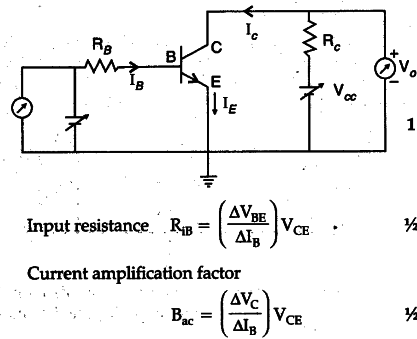# Draw a circuit diagram of a transistor amplifier in CE configuration

Draw a circuit diagram of a transistor amplifier in CE configuration.
Define the terms : (i) Input resistance and (ii) Current amplification factor. How are these determined using typical input and output characteristics ?The value of input resistance is determined from the slope of verses plot at constant
The value of current amplification factor is obtained from the slope of collector Ic verses plot using different values of IB.
(If a student uses typical charateristics is determine these values, full credit of one mark should be given)